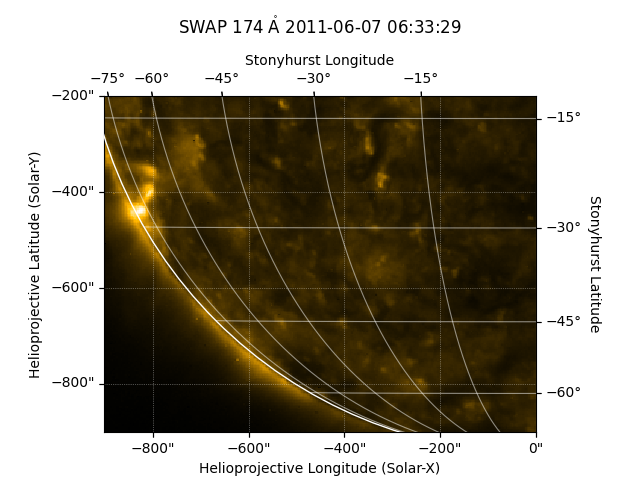# Cropping a Map#

How to crop a map by using submap.

import matplotlib.pyplot as plt

import astropy.units as u
from astropy.coordinates import SkyCoord

import sunpy.data.sample
import sunpy.map


swap_map = sunpy.map.Map(sunpy.data.sample.SWAP_LEVEL1_IMAGE)


To crop the data you create a submap, specifying the top right and bottom left as SkyCoord objects.

top_right = SkyCoord(0 * u.arcsec, -200 * u.arcsec, frame=swap_map.coordinate_frame)
bottom_left = SkyCoord(-900 * u.arcsec, -900 * u.arcsec, frame=swap_map.coordinate_frame)
swap_submap = swap_map.submap(bottom_left, top_right=top_right)

INFO: Missing metadata for solar radius: assuming the standard radius of the photosphere. [sunpy.map.mapbase]


Let’s plot the results.

fig = plt.figure()
image = swap_submap.plot(axes=ax)
swap_submap.draw_limb(axes=ax)
swap_submap.draw_grid(axes=ax)

# Make some room and put the title at the top of the figure
ax.set_position([0.1, 0.1, 0.8, 0.7])INFO: Missing metadata for solar radius: assuming the standard radius of the photosphere. [sunpy.map.mapbase]